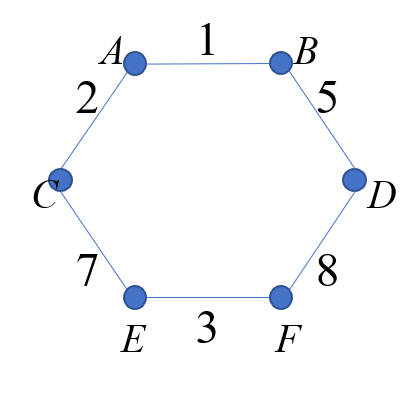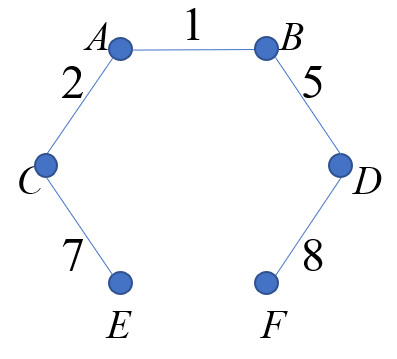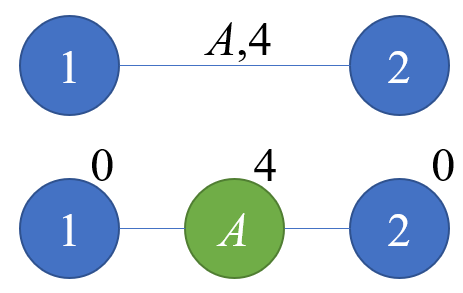2019-01-03 19:34:40

## 题解：## Code：

#include<cstdio>
#include<cstring>
#include<algorithm>
#define ls ch[k]
#define rs ch[k]
#define which(k) (ch[fa[k]]==k)
#define isroot(k) (ch[fa[k]]!=k&&ch[fa[k]]!=k)
using std::max;
int ch,fa;
int key,sum,lazy;
void maintain(int k)
{
sum[k]=max(max(sum[ls],key[k]),sum[rs]);
}
void pushdown(int k)
{
if(lazy[k])
{
int tmp=ls;
ls=rs;
rs=tmp;
lazy[k]=0;
lazy[ls]^=1;
lazy[rs]^=1;
}
}
void Rotate(int k)
{
int y=fa[k];
if(!isroot(y))
ch[which(y)][fa[y]]=k;
bool d=which(k);
fa[k]=fa[y];
fa[y]=k;
ch[d][y]=ch[!d][k];
fa[ch[d][y]]=y;
ch[!d][k]=y;
maintain(y);
maintain(k);
}
int stk,tp=0;
void splay(int k)
{
int x=k;
while(!isroot(x))
{
stk[++tp]=x;
x=fa[x];
}
stk[++tp]=x;
while(tp)
pushdown(stk[tp--]);

while(!isroot(k))
{
int y=fa[k];
if(!isroot(y))
Rotate(which(k)^which(y)?k:y);
Rotate(k);
}
}
void access(int k)
{
for(int x=k,y=0;x;y=x,x=fa[x])
{
splay(x);
ch[x]=y;
maintain(x);
}
}
void makeroot(int k)
{
access(k);
splay(k);
lazy[k]^=1;
}
void split(int x,int y)
{
makeroot(x);
access(y);
splay(y);
}
{
makeroot(x);
fa[x]=y;
}
void cut(int x,int y)
{
makeroot(x);
access(y);
splay(y);
fa[x]=ch[y]=0;
}
int Find(int k,int x)
{
if(key[k]==x)
return k;
if(sum[ls]==x)
return Find(ls,x);
return Find(rs,x);
}
int f,U,V,op;
int n,m,q;
struct edge
{
int x,y,v,ava;
edge(){ava=1;}
friend bool operator <(edge a,edge b)
{
return a.v<b.v;
}
}e;
int s;
int Find(int x)
{
if(s[x]!=x)
return s[x]=Find(s[x]);
return x;
}
void Union(int x,int y)
{
s[Find(x)]=Find(y);
}
void mst()
{
for(int i=1;i<=n;++i)
s[i]=i;
for(int i=1;i<=m;++i)
if(e[i].ava&&Find(e[i].x)!=Find(e[i].y))
{
Union(e[i].x,e[i].y);
}
}
int ans;
int main()
{
scanf("%d%d%d",&n,&m,&q);
for(int i=1;i<=m;++i)
scanf("%d%d%d",&e[i].x,&e[i].y,&e[i].v);
std::sort(e+1,e+1+m);
for(int i=1;i<=m;++i)
{
f[e[i].x][e[i].y]=f[e[i].y][e[i].x]=i;
key[n+i]=e[i].v;
}
for(int i=1;i<=q;++i)
{
scanf("%d%d%d",&op[i],&U[i],&V[i]);
if(op[i]==2)
e[f[U[i]][V[i]]].ava=0;
}
mst();
int cnt=0;
for(int i=q;i>=1;--i)
{
split(U[i],V[i]);//V[i]是根
if(op[i]==1)
ans[++cnt]=sum[V[i]];
else
{
int t=Find(V[i],sum[V[i]]);
if(key[f[U[i]][V[i]]+n]<sum[V[i]])
{
cut(e[t-n].x,t);
cut(e[t-n].y,t);
}